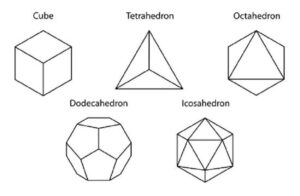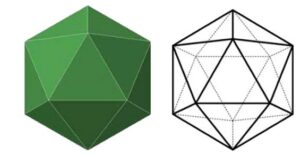# Triangles, Icosahedron and the 5 Regular Solids

An icosahedron is one of the 5 regular solids, and it’s made of equilateral triangles with twenty sides. I have been fascinated by regular solids, and the Icosa is the one that arouses my curiosity the most. If you love geometric shapes and their application, walk with me.

### Triangles

“A triangle is a pattern where each side stabalises the opposite angle with minimum effort” – Bucky Fuller.

A Triangle is known to be the strongest shape. Any weight placed on it is evenly distributed on its 3 sides. Unlike other shapes like squares, if pressure is applied to one side of the triangle, it shifts into a rhombus. No matter the pressure applied to a triangle, it will absorb it and remain rigid.

#### There are three types of triangles:

1. Equilateral triangle – three sides of equal length
2. Isosceles triangle – two sides of equal length
3. Scalene triangle – no sides of equal length

We can get other shapes from the triangle, like the Tetrahedron, Octahedron and Icosahedron.

The Tetrahedron is the basic structural system – 3 Triangles around each corner.

The Octahedron has 4 Triangles around each corner, while the Icosahedron has 5 Triangles around each corner.

Some of the applications of this system are in the architecture of truss bridges, geodesic domes, A-frames and other structures.### Icosahedron and the 5 Regular Solids

The Icosahedron, Tetrahedron, Cube, Octahedron and Dodecahedron are the only Five Regular Solids (Polyhedra) possible in Nature’s Coordinating System. For a solid to be Regular, all its faces should be identical, and all the vertices should lie on a sphere.

These five regular solids are also known as Platonic solids, as they were first given Nature’s elemental characteristics by the Athenian philosopher Plato in his book Timaeus in 360 BC.

According to Plato, each solid represents an element in nature as follows;

1. Tetrahedron – Fire
2. Cube – Earth
3. Octahedron – Air
4. Icosahedron – Water
5. Dodecahedron – Universe

It is also argued that Pythagoras of Samos, who greatly influenced Plato’s philosophies, first discovered solids. The Icosahedron is the basis for the Geodesic Dome.

In the next article, I’ll share my progress in constructing a 1V Geodesic Dome based on the calculations of Bucky Fuller.